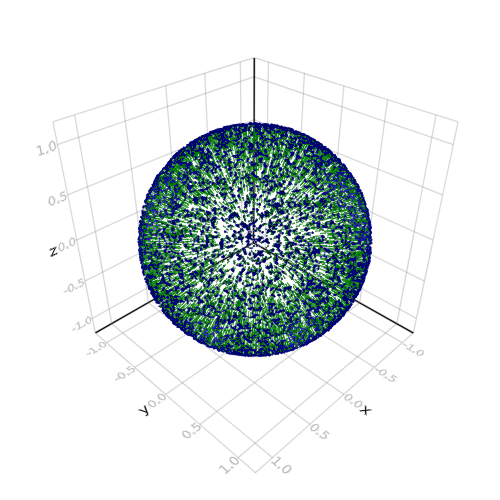## Arrows 3D

```
using LinearAlgebra

using AbstractPlotting

function SphericalToCartesian(r::T,θ::T,ϕ::T) where T<:AbstractArray
x = @.r*sin(θ)*cos(ϕ)
y = @.r*sin(θ)*sin(ϕ)
z = @.r*cos(θ)
Point3f0.(x, y, z)
end
n = 100^2 #number of points to generate
r = ones(n);
θ = acos.(1 .- 2 .* rand(n))
φ = 2π * rand(n)
pts = SphericalToCartesian(r,θ,φ)
arrows(pts, (normalize.(pts) .* 0.1f0), arrowsize = 0.02, linecolor = :green, arrowcolor = :darkblue)

```